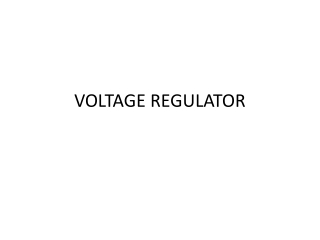DownloadDownload PresentationVOLTAGE REGULATOR

# VOLTAGE REGULATOR

Télécharger la présentation## VOLTAGE REGULATOR

- - - - - - - - - - - - - - - - - - - - - - - - - - - E N D - - - - - - - - - - - - - - - - - - - - - - - - - - -
##### Presentation Transcript

1. VOLTAGE REGULATOR

2. Voltage Regulator • Zener diode is a voltage regulator device because it is able to fix the output voltage at a constant value (DC voltage). • RS is to limit the zener current, IZ so that it is less than the maximum current, IZM (to avoid the zener diode from broken).

3. Zener as Regulator A simple regulator circuit A regulator circuit with load resistance

4. Voltage Regulator • How to determine whether the zener acts as a regulator or not?? • Use Thevenin Theorem • See example • If VTH<VZ, regulation does not occur.

5. Voltage Regulator…. Referring to zener I-V charateristic curve, if the voltage across the zener diode zener is between 0-VZ, the zener diode is operating in the reverse bias region, thus it DOES NOT functioned as a regulator. VTH must at least the same value as VZ(VTH VZ) so that the diode CAN function as a voltage regulator because it is operating in reverse breakdown region.

6. Zener I-V Charateristic Reverse biased region Reverse breakdown region

7. Zener Diode Regulator In this simple illustration of zener regulation circuit, the zener diode will “adjust” its impedance based on varying input voltages and loads (RL) to be able to maintain its designated zener voltage. Zener current will increase or decrease directly with voltage input changes. The zener current will increase or decrease inversely with varying loads. Again, the zener has a finite range of operation.

8. Thevenin Equivalent Circuit

9. Examples of zener as voltage regulator

10. Cases in Zener Regulator Circuits

11. Zener Diode • Three types of Zener analysis • Fixed VS and RL • Fixed VS and variable RL • Variable VS and fixed RL

12. Zener Diode - Fixed VS and RL • The applied dc voltage is fixed, as the load resistor. • The analysis : • Determine the state of the Zener diode by removing it from the network and calculating the voltage across the resulting open circuit.

13. Zener Diode 2. Substitute the appropriate equivalent circuit and solve for the desired unknowns. - For the on state diode, the voltages across parallel elements must be the same. VL=VZ The Zener diode current is determined by KCL: IZ = IR – IL The power dissipated by the Zener diode is determined by: PZ = VZ IZ - For the off state diode, the equivalent circuit is open-circuit.

14. Zener Diode - Fixed VS and Variable RL Step 1- get the RLmin so that zener is on. Rs + Vs - - if RL ≥ RLmin, zener diode ‘on’, so that VL=VZ Step 2: Calculate the IZ using KCL: 2 condition 1. If RLmin , then ILmax and IZmin because of constant I1 2. If RLmax, then ILmin and IZmax

15. Zener Diode ; Izmax taken from data sheet Izmin = 0, if not given or and Where

16. Zener Diode - Variable VS and fixed RL Step 1- get the VSmin so that zener is on. Rs + Vs - if VS ≥ VSmin, zener diode will ‘on’, so that VL=VZ Step 2: Calculate the IZ using KCL: 2 condition 1. if VSmin , then I1min and IZmin because of constant IL 2. if VSmax, thenI1max and IZmax

17. Zener Diode ; Izmax= Pzmax/Vz or and where

18. IC Regulator The 78xx (also sometimes known as LM78xx) series of devices is a family of self-contained fixed linear voltage regulator IC. The 78xx family is a very popular choice for many electronic circuits which require a regulated power supply, due to: i. ease of use and ii. Low cost.

19. 78xx and 79xx IC When specifying individual ICs within this family, the xx is replaced with a two-digit number, which indicates the output voltage the particular device is designed to provide (for example, the 7805 has a 5 volt output, while the 7812 produces 12 volts). The 78xx line are positive voltage regulators, meaning that they are designed to produce a voltage that is positive relative to a common ground. There is a related line of 79xx devices which are complementary negative voltage regulators. 78xx and 79xx ICs can be used in combination to provide both positive and negative supply voltages in the same circuit, if necessary.